# HC Verma Solutions Class 12 Chapter 10 Electric Current In Conductors

HC Verma Solutions Class 12 Chapter 10 Electric Current in Conductors exposes students to the right answers for all the different levels and types of questions discussed in the chapter. The solutions that are provided here have been put together by experts to further help students boost their scoring potential in the exams. Therefore students can make use of the solutions to practice solving questions and at the same time learn the right answers as well as find new ways to solve problems.

## Electric Current In Conductors Key Topics

In chapter 10, students will several topics that they should be familiar with. Some key topics include;

• Drift Speed
• Ohm’s Law
• Temperature Dependence of Resistivity
• Battery and EMF
• Kirchoff’s Law
• Ammeter and Voltmeter
• Atmospheric Electricity
• Energy Transfer in an Electric Current

Students will further learn about the mathematical relationship between voltage, resistance, and electric current. Using HC Verma Solutions will be beneficial for students as they can study more efficiently and solve even the complex questions easily. Class 12 students can use this study material for various competitive exams like JEE as well.

## Class 12 Important Questions In Chapter 10

1. Do you think that the work done by a battery is always equal to the thermal energy generated in electrical circuits? State the effect of the capacitor when it is connected in the circuit?

2. A lot of times people tend to say “a current is going through the wire”. What do you think flows or moves through the wire, the current or the charge?

3. A fan having copper winding in its motor uses less power in comparison to fans having aluminium winding. Explain this statement.

4. What does the product of conductivity and resistivity of a cylindrical conductor depend upon?

(a) material (b) area of cross-section (c) temperature(d) Does not depend on these factors

5. Why should the net resistance of an ammeter be small?

(a) it does not appreciably change the current to be (b) it does not draw excessive current (c) it does not get overheated (d) it can measure large currents

6. When a resistor is connected to a battery which is heated due to the current what quantities will change?

(a) number of free electrons (b) resistance (c) resistivity (d) drift speed

## HC Verma Solutions Vol 2 Electric Current in Conductors Chapter 10

Question 1: The amount of charge passed in time t through a cross-section of a wire is Q(t) = At2 + Bt + C.

(a) Write the dimensional formulae for A, B and C.

(b) If the numerical values of A, B and C are 5, 3 and 1 respectively in S.I. units, find the value of the current at t = 5 s.

Solution:

Amount of the charge: Q(t) = At2 + Bt + C

On comparing the dimensions of each term with the dimension of the charge, we have

Q = At2

A = Q/t2 = It/t2 = l/t

=> A = [AT-1]

Again, Bt = Q

=> B = Q/t = I = [A]

Also, Q = C

=> C = [AT]

(b) I = dQ/dt = d/dt(At2+Bt+C)

= 2At + B

Here A = 5, B = 3 and t = 5s

=> I = 53 A

Question 2: An electron gun emits 2.0 × 1016 electrons per second. What electric current does this correspond to?

Solution:

In time, t = 1s, seconds 2.0 × 1016 electrons are emitted.

Total charge flowing from the gun = q = ne

= 2 x 1016 x 1.6 x 10-19 C

= 3.2 x 10-3 C

Corresponding current = i = q/t = [3.2×10-3]/1 = 3.2 x 10-3 A

Question 3: The electric current existing in a discharge tube is 2.0 μA. How much charge is transferred across a cross-section of the tube in 5 minutes?

Solution:

Time (t) for which charge transfer = 5 min = 300s

Electric current in the tube = 2.0 μA

Charge transferred across the cross-section in 300s = q = i t = 2 x 300 = 6 x 10-4 C

Question 4: The current through a wire depends on time as

i = i0 + αt,

Where i0 = 10 A and α = 4 As–1. Find the charge crossed through a section of the wire in 10 seconds.

Solution:

The current through a wire depends on time

i = i0 + αt …(1)

Where, i0 = 10A, t = 10s, and a = 4 As–1

t = time for which current passes

For the given expression of current, charge q is,

q = ∫i dt

From 0s = 0 to ts and using (1), we get

q = i0 t + at2/2

= 10 x 10 + (4×10-4)/2

= 300 C

Therefore, charge crossed through a section of the wire in 10 seconds is 300C.

Question 5: A current of 1.0 A exists in a copper wire of cross-section 1.0 mm2. Assuming one free electron per atom calculate the drift speed of the free electrons in the wire. The density of copper is 9000 kg m–3.

Solution:

Cross section of the wire, A= 1mm2 = 10-6 m2

Density of Copper, ρ = 9000 kg m–3

current in the wire, i = 1 A

We know, i = nAeVd …..(1)

Which is the current due to ‘n’ freely bounded electrons per unit volume with a drift speed ‘Vd

and e = charge of the electron = 1.6×10-19C.

First find “n”:

we know, 63.5 grams of Copper have 6.022×1023 of atoms. So ‘m’ Kilograms have,

[6.022×1023 x mKg]/[63.5×10-3 Kg]

Mass in terms of density, m = unit volume x ρ

The number of free electrons = [6.022×1023 x 9000Kgm-3]/[63.5×10-3 Kg] [by dividing expression by unit volume]

Now, solving above we have n = 8.535 x 1028

(1)=> Vd = i/nAe

Substituting the known values, we have

Vd = 7.32 x 10-5 ms-1 = 0.074 mms-1

Question 6: A wire of length 1 m and radius 0.1 mm has a resistance of 100 Ω. Find the resistivity of the material.

Solution:

We know, the resistivity, ρ, of a wire with cross-sectional area A and length l,

ρ = RA/l …(1)

Where : R is the resistance offered by the wire.

Area of cross-section of the wire = A = π r2 = π (0.1 x 10-3 m) 2 = 3.14 x 10-8 m2

R = 100Ω and l = 1m (Given)

(1)=> ρ = 3.14 x 10-6 Ω m

Question 7: A uniform wire of resistance 100 Ω is melted and recast in a wire of length double that of the original. What would be the resistance of the wire?

Solution:

Let R1 = Initial Resistance of the wire = 100 Ω

l1= Initial length of wire and l2 = Final length of wire

Therefore, l2 = 2 l1

We know, resistance of a wire = R = ρl/A ….(1)

We know that the volume remains same after the recast in a wire.

So, A1 l1 = A2 x l2

using l2 = 2 l1

=> A2 = A1/2

Initial and final Resistance of the wire: R1 = ρ1l1/A1 and R2 = ρ2l2/A2

Since ρ1 = ρ2.

=> R2/R1 = l2A2/l1A1 = 2l1A1/l1(A2/2)

=> R2 = 4R1 = 4 x 100 = 400Ω

Question 8: Consider a wire of length 4 m and cross-sectional area 1 mm2 carrying a current of 2 A. If each cubic meter of the material contains 1029 free electrons, find the average time taken by an electron to cross the length of the wire.

Solution:

Current through the wire = I = 2A

Area of cross-section = A = 1 mm2 = 10-6 m2

Number of free electron per unit volume= n = 1029

Length of wire = l= 4m

We know, Vd = i/nAe

Vd = drift velocity

Here, e is the charge of 1 electron= 1.6×10-19 C

VD in terms of length and time, VD = l/t

=> i/nAe = l/t

t = nAel/i

= [1029 x 10-6 x 1.6×10-19x4]/2 = 32000 s = 8.89 hours

Therefore, average time taken by an electron to cross the length of the wire is approximately 8.9 hours.

Question 9: What length of a copper wire of cross-section area 0.01 mm2 will be needed to prepare a resistance of 1 kΩ? Resistivity of copper = 1.7 × 10–8 Ω m.

Solution:

Resistivity of wire = 1000Ω

Resistivity of copper, = 1.7 × 10-8 Ω m

Area of cross-section = A=0.01 mm2 = 0.01×10-6 m2

We know, R = ρl/A

or l = AR/ρ

= [(0.01×10-6)x1000]/[1.7×10-8]

= 588.23 m

= 0.6 km (approx)

Question 10: Figure shows conductor of length ℓ having a circular cross-section. The radius of cross-section varies linearly from a to b. The resistivity of the material is ρ. Assuming that b – a << ℓ, find the resistance of the conductor.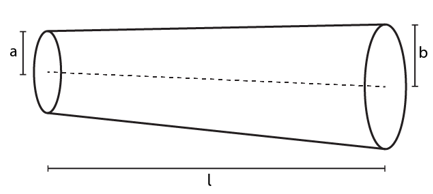Solution:

Resistance of a cylinder with length ‘l’ and area of cross-section A is,

R = ρl/A

From the figure, relation connecting x and y: (b-a)/l = (y-a)/x

or x(b-a) = l(y-a)

Differentiating above expression w.r.t. x and rearranging, we have

dx = ldy/(b-a) …(1)

Let us assume that, cone is made up of an infinite number of cylinders with length ‘dx’.

So for that element, resistance dR:

dR = ρdx/A

Again, resistance for an element with a radius ‘y’ at x distance from left with length dx:

dR = ρdx/A In this case, A = πy2

=> dR = ρdx/πy2 …(2)

To get total resistance (R) integrate above expression form the range a to b and using (1), we have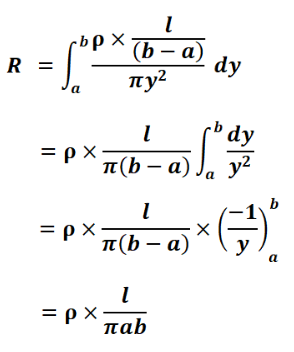Which is the resistance of the truncated cone.

Question 11: A copper wire of radius 0.1 mm and resistance 1 kΩ is connected across a power supply of 20 V.

(a) How many electrons are transferred per second between the supply and the wire at one end?

(b) Write down the current density in the wire.

Solution:

r= 0.1mm = 0.1×10-3m

R= 1000Ω and V = 20V

(a) We know, ne/t = V/R

[using relations, I = V/R, I = q/t and q = ne]

n = Vt/eR

Where, n = number of electrons passed in 1s through the copper wire with resistance 1000Ω

For time t= 1s

n = [20×1]/[1.6×10-19x1000]

= 1.25 x 1017 electrons

(b)

area of cross-section of a wire = A = π r2

= π (0.1×10-3)2 = 3.1415 x 10-8 m2

Therefore, I = 20/1000 = 0.02 A

[using relation, I = V/R]

Now, current density, J = I/A

=> J = 0.02/[3.1415 x 10-8]

= 6.366×105 Am-2

Question 12: Calculate the electric field in a copper wire of cross-section area 2.0 mm2 carrying a current of 1 A. The resistivity of copper = 1.7 × 10–8 Ω m.

Solution:

Area of cross-section of wire = A=2.0 mm2 = 2×10–6 m2

Current flowing through the wire = I = 1A

Resistivity of copper = ρ= 1.7 × 10–8 Ω m

We know, the Electric field, E = V/l …(1)

Where l is the distance over which potential difference V

Again from Ohm’s law, we have R = ρl/A …(2)

[relation connecting resistance, R and resistivity, ρ]

Where ‘l’ is the length of the wire.

And, potential difference, V is V = IR ….(3)

using (2) and (3) relations, we have

E = Iρl/Al = Iρ/A

= [1 x 1.7×10-8]/[2×10-6]

=> E = 0.0085 m-1

Question 13: A wire has a length of 2.0 m and a resistance of 5.0 Ω. Find the electric field existing inside the wire if it carries a current of 10 A.

Solution:

Length of the wire= l = 2m

Resistance of the wire= R = 5 Ω

Current passing through the wire= I = 10A

We know, the Electric field, E = V/l …(1)

Where l is the distance over which potential difference V

We know that the potential difference, V = IR = 50 V

[Using given values]

=> E = 50/2 = 25 V/m

Question 14: The resistances of an iron wire and a copper wire at 20°C and 3.9 Ω and 4.1 Ω respectively. At what temperature will the resistances be equal? Temperature coefficient of resistivity for iron is 5.0 × 10–3 K–1 and for copper it is 4.0 × 10–3 K–1. Neglect any thermal expansion.

Solution:

The resistances of an iron wire and a copper wire at 20°C and 3.9 Ω and 4.1 Ω respectively.

R(Fe, i) at 20°C = 3.9 Ω and R(Cu, i) at 20°C = 4.1 Ω

Initial temperature of both the wires = Ti = 20°C

Temp coefficient of resistivity for copper = αCu = 4.0 × 10–3 K–1

Temp coefficient of resistivity for iron = αFe = 5.0 × 10–3 K–1

Relation connecting the resistance with the change in temperature:

Rf = Ri(1 + αΔT) …(1)

Where α is the Temperature coefficient of resistivity and ∆T is the change in temperature

and ∆T = Tf – Ti

Now,

Final resistance of iron wire: RFe,f = RFe,i(1 + αFe ΔT)

= 3.9 x(1 + (5×10-3) ΔT)

Final resistance of copper wire: RCu,f = RCu,i(1 + αCu ΔT)

= 4.1 x(1 + (4×10-3) ΔT)

At the final temperature, it is given that the resistance of both the wires are same.

=> RFe,f = RCu,f

3.9 x(1 + (5×10-3) ΔT) = 4.1 x(1 + (4×10-3) ΔT)

On solving above, we have

ΔT = 64.5oC

Now, ∆T = Tf – Ti

=> Tf = 64.5oC + 20oC = 84.5oC, at this temperature resistances are equal.

Question 15: The current in a conductor and the potential difference across its ends are measured by an ammeter and a voltmeter. The meters draw negligible currents. The ammeter is accurate but the voltmeter has a zero error (that is, it does not read zero when no potential difference is applied). Calculate the zero error if the readings for two different conditions are 1.75 A, 14.4 V and 2.75 A, 22.4 V.

Solution:

Ammeter reading of current in the 1st case = i1 =1.75A

Voltmeter reading for current i1, V1=14.4V

Ammeter reading of current in the 2nd case= i2 = 2.75A

Voltmeter reading for current i2, V2 = 22.4V

Let V be the magnitude of zero error in the voltmeter reading.

Under given conditions, the resistance of the wire will remain same. So

R1 = R2

=> I1/I2 = (V1-V)/(V2-V)

[using relation, V = IR]

=> 1.75/2.75 = (14.4-V)/(22.4-V)

=> 7/11 = (14.4-V)/(22.4-V)

Solving above for V, we get

V = 0.4 V

Therefore, the zero error associated with the measurement is 0.4V. This value can be -ve or +ve, these values just indicate that the needle of the voltmeter is the left or right of the zero if zero voltage applied across the voltmeter.

Question 16: Figure shows an arrangement to measure the emf ϵ and internal resistance r of a battery. The voltmeter has a very high resistance and the ammeter also has some resistance. The voltmeter reads 1.52 V when the switch S is open. When the switch is closed the voltmeter reading drops to 1.45 V and the ammeter reads 1.0 A. Find the emf and the internal resistance of the battery.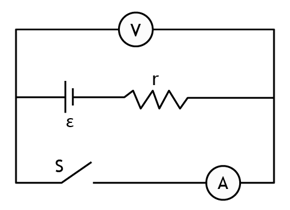Solution: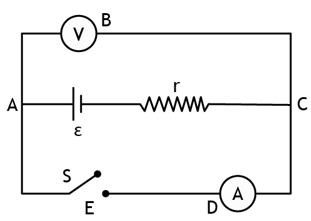Case 1: When switch is open, the current would circulate through loop ABCA only. Since the internal resistance is very small compared to the resistance of the voltmeter, the voltage drop occurs entirely at the voltmeter. This voltage drop is measured in the measuring device and almost corresponds to the emf of the cell.

=> e.m.f of cell= volt meter reading.

So, emf = 1.52 V

Case 2: When the switch is closed, current ‘i’ will flow through the loop ACDEA. The voltmeter shows the potential drop across the cell and the internal resistance together. Let us find the internal resistance of the cell,

V= ε – ir

Where V = Volt meter reading = 1.45V, ϵ = 1.52V and i = Ammeter reading = 1A

or r = (ε-V)/i

or r = [1.52-1.45]/1 = 0.07 Ω

Question 17: The potential difference between the terminals of a battery of emf 6.0 V and internal resistance 1 Ω drops to 5.8 V when connected across an external resistor. Find the resistance of the external resistor.

Solution: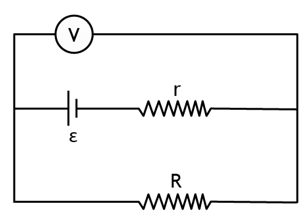The current flowing through the internal and external resistance are the same.

We know, The voltage across the cell will be equal to that across the external resistance.

So, E – ir = iR = V

In this case, E – ir = V

6V – i x 1Ω = 5.8 V

or i = 0.2 A

Also, we know V = iR

0.2 A x R = 5.8 V

=> R = 29 Ω, is the resistance of the external resistor.

Question 18: The potential difference between the terminals of a 6.0 V battery is 7.2 V when it is being charged by a current of 2.0 A. What is the internal resistance of the battery?

Solution:

The current flowing through the internal and external resistance are the same.

We know, The voltage across the cell will be equal to that across the external resistance.

So, E – ir = iR = V

In this case, r = (V-E)/i

Given: V = 7.2V ; E = 6V and i = 2A

=> r = 0.6Ω, is the internal resistance of the cell.

Question 19: The internal resistance of an accumulator battery of emf 6 V is 10 Ω when it is fully discharged. As the battery gets charged up, its internal resistance decreases to 1Ω. The battery in its completely discharged state is connected to a charger which maintains a constant potential difference of 9V. Find the current through the battery

(a) just after the connections are made and

(b) after a long time when it is completely charged.

Solution:

(a) When the battery is being charged, the net emf, Enet across the resistance, r1 is

Enet = Ec – E

E = emf of the battery = 6V

Ec = Potential difference provided by the charger = 9V

=> Enet = 3 V

Also, we know V = ir1

or i = V/r1 = 3/10 = 0.3 A

Given: The internal resistance of the battery, r1 when discharged = 10Ω

(b) When the battery is completely charged, the internal resistance r2 will be 1Ω.

Now, i = 3/1 = 3 A

Therefore, current through the internal resistance while charging and after completely charged are 0.3A and 3A respectively.

Question 20: Find the value of i1/i2 in figure if

(a) R = 0.1Ω, (b) R = 1 Ω (c) R = 10 Ω.

Note from answers that in order to get more current from a combination of two batteries they should be joined in parallel if the external resistance is small and in series if the external resistance is large as compared to the internal resistances.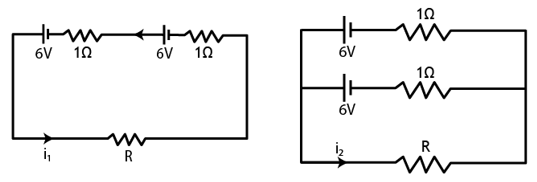Solution:

(a)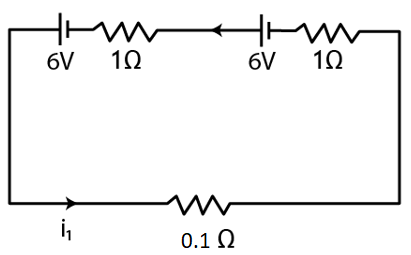Applying Loop rule, we have

6 V + 6V = i1 x 0.1Ω + i1 x 1Ω + i1 x 1Ω

[Using relation, V = iR]

or 12 V = i1 2.1 Ω

or i1 = 5.71 A

Now, from figure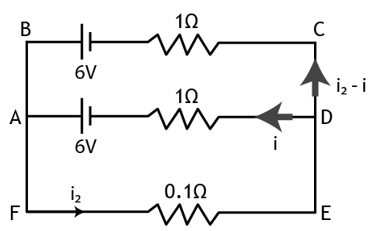Current i passes through the middle branch and i2 – i will pass through the upper branch, apply loop rule in AFEDA

6 V = i2 x 0.1Ω + i x 1Ω

or i = 6 – 0.1 i2

Again, apply loop rule in ADCBA

-6V – i x 1Ω + (i2 – i) x 1Ω + 6V = 0

or i2 = 2i

Using results of both the loops,

i2 = 10 A and i1 = 5.71 A

Their ratio: i1/ i2 = 5.71 A/10 A = 0.57

(b)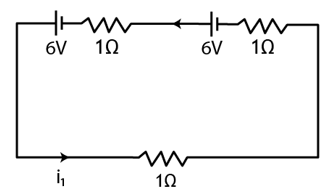Applying Loop rule,

6 V + 6V = i1 x 1Ω + i1 x 1Ω + i1 x 1Ω

12V/3 Ω = i1

Or i1 = 4 A

From second figure,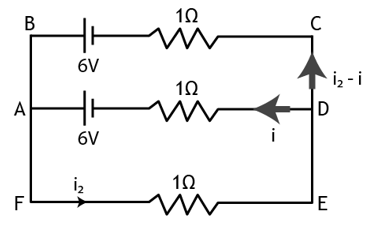By applying loop rule in AFEDA,

6 V = i2 x 1Ω + i x 1Ω

or i = 6 – i2

Again, apply loop rule in ADCBA

-6V – i x 1Ω + (i2 – i) x 1Ω + 6V = 0

or i2 = 2i

Using results of both the loops,

i2 = 4 A and i1 = 4 A

Their ratio: i1/ i2 = 4 A/4 A = 1

(c)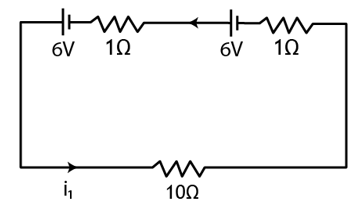Applying Loop rule,

6 V + 6V = i1 x 10Ω + i1 x 1Ω + i1 x 1Ω

12V/12 Ω = i1

Or i1 = 1 A

From second figure,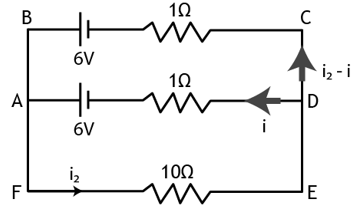By applying loop rule in AFEDA,

6 V = i2 x 10Ω + i x 1Ω

or i = 6 – 10 i2

Again, apply loop rule in ADCBA

-6V – i x 1Ω + (i2 – i) x 1Ω + 6V = 0

or i2 = 2i

Using results of both the loops,

i2 = 0.57 A and i1 = 1 A

Their ratio: i1/ i2 = 4 A/0.57 A = 1.75

Question 21: Consider N = n1n2 identical cells, each of emf ϵ and internal resistance r. Suppose n1 cell are joined in series to form a line and n2 such lines are connected in parallel. The combination drives a current in an external resistance R.

(a) Find the current in the external resistance.

(b) Assuming that n1 and n2 can be continuously varied, find the relation between n1, n2, R and r for which the current in R is maximum.

Solution:

(a) From given, Total emf in every branches are the same.

Let us calculate for one branch:

When n1 cells each with emf ‘E’ are connected in series.

Total emf, Enet is Enet = n1 E

The resistance of n1 cells each with resistance ‘r’ in series is Ro = n1 r

Total resistance for such n2 number of branches, connected in parallel:

Reff = n1r/n2

Total net resistance when whole setup is connected to an external resistance R. will be,

Rnet = n1r/n2 + R

Let i be the current through the external resistor i.e. i = Enet/Rnet

or i = n1n2E/[n1r+n2R] …(1)

(b)

To get the minimum current through the resistor R, the denominator of equation (1) must be minimum.

Now, n1r+n2R = (√ n1r – √ n2R)2 + 2 √ (n1n2r)

n1r + n2R is only minimum when √ n1r = √ n2R

or n1r = n2R

Therefore, i is maximum when n1r = n2R

Question 22: A battery of emf 100 V and a resistor of resistance 10 kΩ are joined in series. This system is used as a source to supply current to an external resistance R. If R is not greater than 100 Ω, the current through it is constant up to two significant digits. Find its value. This is the basic principle of a constant-current source.

Solution: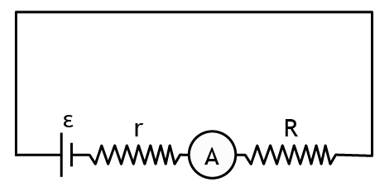We know that, current i for a series resistor connection: io = E/r

Given: E = 100 V and r= 10kΩ = 10000kΩ

i = 0.01 A

Consider R as 2 Ω

i = 100V/Rtot

Where Rtot is the effective resistance across the battery.

Rtot = r + R,

So i = 100V/(r+R) = 100/(10000+2) = 0.01 A (approx.)

Similarly, on putting R=100Ω , the highest possible, we have

i = 100V/(r+R) = 100/(10000+100) = 0.01 A (approx.)

We can see that up to R = 100 Ω, the current does not change up to two significant digits

Question 23: If the reading of ammeter A1 in figure below, what will the ammeters A2 and A3 read? Neglect the resistances of the ammeters.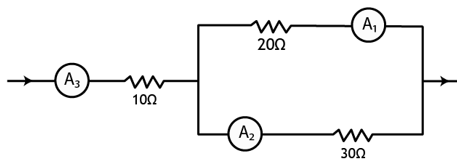Solution:

Current through A1 ammeter, i – i1 = 2.4A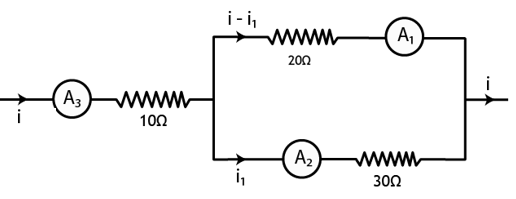The current passing through Ammeter A3 will divide into two branches. To find the current in both the branches by equating the voltage in each branches.

(i – i1) x 20Ω = i1 x 30Ω

Put i – i1 = 2.4A, here i1 goes through ammeter A2

i1 = [2.4×20]/30 = 1.6 A

Total current i, passing through A3

i = (i-i1) + i1 = 2.4A + 1.6A = 4A

The current through A2 and A3 are 1.6A and 4A.

Question 24: The resistance of the rheostat shown in figure is 30 Ω. Neglecting the meter resistance, find the minimum and maximum currents through the ammeter as the rheostat is varied.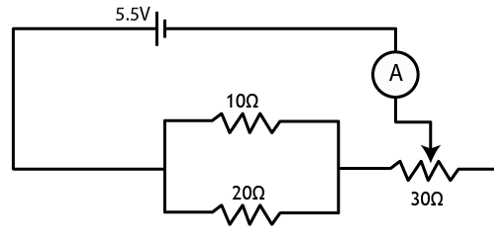Solution:

The 10 Ω and 20 Ω connected parallel to each other. The effective resistance, Reff between 10 Ω and 20 Ω will be,

Reff = [10×20]/[10+20] = 6.667Ω

The minimum current will be marked when the total resistance is maximum, which happens when rheostat resistance, R=30Ω.

So, current = i = E/R_tot and Rtot = Reff + 30Ω = 36.667Ω

The minimum current, imin = 5.5V/36.667Ω = 0.15A

Similarly, maximum current, imax can be find when Rheostat resistance R is minimum, R = 0Ω.

Rtot = Reff + 0Ω = 6.667Ω

Therefore, imax = E/Rtot = 5.5V/6.667Ω = 0.83A

The current in the ammeter vary from 0.15A to 0.83A

Question 25: Three bulbs, each having a resistance of 180 Ω, are connected in parallel to an ideal battery of emf 60 V. Find the current delivered by the battery when

(a) all the bulbs are switched on,

(b) two of the bulbs are switched on and

(c) only one bulb is switched on.

Solution: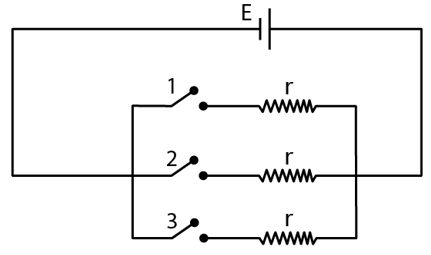(a) When all the switches are closed. Current will flow equally across each of the resistors. And total current will be the ratio between the potential difference and the effective resistance.

The effective resistance = Reff = r/3

= 180Ω/3

= 60Ω

and current = i = E/Reff = 60V/60Ω = 1 A

(b) Two resistors are connected in parallel.

Effective resistance = Reff = 180Ω/2 = 90Ω

and current = i = E/Reff = 60V/90Ω = 0.67 A

(c) Only one resistor is connected to the battery.

Effective resistance = Reff = r = 180Ω

and current = i = E/Reff = 60V/180Ω = 0.33 A